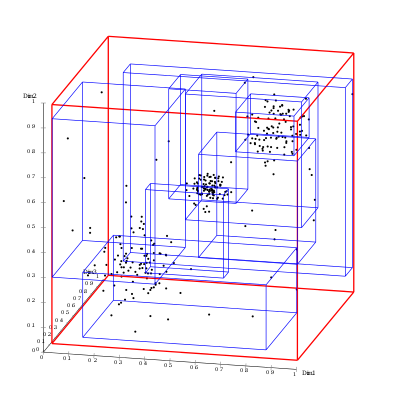# RTree Visualization

Is there any way to visualize RTree method coming from RhinoCommon similar to original Wikipedia description?I don’t think so with RhinoCommon, because you don’t have access to the tree nodes. In C++ it can be done.

Thanks Menno I ll give a go to C++

The docs are here

https://developer.rhino3d.com/api/cpp/class_o_n___r_tree.html

Is there any sample in McNeel github page as well?

Not that I can see. I know I have some code that uses it on my company laptop, I can post something on Monday.

Thanks;)

Here are a few sa,[;es:

cmdSampleRTree.cpp

cmdSampleRTreeTest.cpp

– Dale

Thank you, do you think c++ sdk allows to vizualize the rtee node hierarchy? Would it be possible to tell me which property I should look at?

Below you find a working example, maybe @dale can put it up on github?

``````bool HandleTreeNode(const ON_RTreeNode* node, ON_SimpleArray<ON_BoundingBox>& boxes)
{
// a tree node contains m_count branches
for (int i = 0; i < node->m_count; ++i)
{
const ON_RTreeBranch& branch = node->m_branch[i];
// each branch has a bounding box in m_rect: this is what we want to see
const ON_RTreeBBox& box = branch.m_rect;
// add the bounding box to the boxes array
ON_3dPoint minPt(box.m_min);
ON_3dPoint maxPt(box.m_max);
ON_BoundingBox bbox(minPt, maxPt);
boxes.Append(bbox);
// if the node is not a leaf node, recurse to the next level
if (!node->IsLeaf())
{
HandleTreeNode(branch.m_child, boxes);
}
}
return true;
}

CRhinoCommand::result CCommandClass::RunCommand( const CRhinoCommandContext& context )
{
CRhinoGetObject gp;

gp.SetCommandPrompt(L"Select points");
gp.SetGeometryFilter( CRhinoGetObject::point_object);
CRhinoGet::result gr = gp.GetObjects(1,0);

// create the R-tree from the input points.
ON_RTree tree;
if (gr == CRhinoGet::result::object)
{
for (int i = 0; i < gp.ObjectCount(); ++i)
{
auto obj = gp.Object(i);
const ON_3dPoint& pt = obj.Point()->point;
tree.Insert(pt, pt, i);
}
}
else
{
return CRhinoCommand::success;
}

ON_SimpleArray<ON_BoundingBox> boxes;
// call the recursive method to extract the boxes,
// starting at the root of the tree
HandleTreeNode(tree.Root(), boxes);

// add the boxes to the document as meshes.
const int nBoxes = boxes.Count();
for (int i = 0; i < nBoxes; ++i)
{
ON_BoundingBox& bbox = boxes[i];

// skip coincident bounding boxes
if (bbox.m_min == bbox.m_max) continue;

// check for planar bounding boxes
bool isPlanar = {false,false,false};
for (int i = 0; i < 3; ++i)
{
if (bbox.m_min[i] == bbox.m_max[i])
{
isPlanar[i] = true;
break;
}
}

// add planar bounding boxes as a mesh face.
ON_SimpleArray<ON_3dPoint> corners;
if (isPlanar)
{
corners.Append(ON_3dPoint(bbox.m_min, bbox.m_min, bbox.m_min));
corners.Append(ON_3dPoint(bbox.m_min, bbox.m_max, bbox.m_min));
corners.Append(ON_3dPoint(bbox.m_min, bbox.m_max, bbox.m_max));
corners.Append(ON_3dPoint(bbox.m_min, bbox.m_min, bbox.m_max));
}
else if (isPlanar)
{
corners.Append(ON_3dPoint(bbox.m_min, bbox.m_min, bbox.m_min));
corners.Append(ON_3dPoint(bbox.m_max, bbox.m_min, bbox.m_min));
corners.Append(ON_3dPoint(bbox.m_max, bbox.m_min, bbox.m_max));
corners.Append(ON_3dPoint(bbox.m_min, bbox.m_min, bbox.m_max));
}
else if (isPlanar)
{
corners.Append(ON_3dPoint(bbox.m_min, bbox.m_min, bbox.m_min));
corners.Append(ON_3dPoint(bbox.m_max, bbox.m_min, bbox.m_min));
corners.Append(ON_3dPoint(bbox.m_max, bbox.m_max, bbox.m_min));
corners.Append(ON_3dPoint(bbox.m_min, bbox.m_max, bbox.m_min));
}

if (corners.Count() > 0)
{
ON_Mesh face;
if (RhinoMeshFace(face, corners))
{
if (face.IsValid())
{
}
}
}
else // add non-planar bounding boxes as boxes.
{
ON_3dPoint meshCorners;

meshCorners = ON_3dPoint(bbox.m_min, bbox.m_min, bbox.m_min);
meshCorners = ON_3dPoint(bbox.m_min, bbox.m_max, bbox.m_min);
meshCorners = ON_3dPoint(bbox.m_max, bbox.m_max, bbox.m_min);
meshCorners = ON_3dPoint(bbox.m_max, bbox.m_min, bbox.m_min);
meshCorners = ON_3dPoint(bbox.m_min, bbox.m_min, bbox.m_max);
meshCorners = ON_3dPoint(bbox.m_min, bbox.m_max, bbox.m_max);
meshCorners = ON_3dPoint(bbox.m_max, bbox.m_max, bbox.m_max);
meshCorners = ON_3dPoint(bbox.m_max, bbox.m_min, bbox.m_max);

ON_Mesh* boxMesh = RhinoMeshBox(meshCorners, 1, 1, 1);

if (nullptr != boxMesh && boxMesh->IsValid())
}
}

return CRhinoCommand::success;
}
``````
3 Likes

Very nice, thank you so muchSince you have experience with C++ an RTree, it seems to give much more freedom.

In C# Rtree is limited to points and bounding boxes.
Do you think it is possible extend C++ RTree to work with oriented-bounding-boxes as well?
Or have you been using C++ RTree with other data-structures rather than point-clouds or boundingboxes?

No, also in C++ the bounding boxes in the datastructure are always axis-aligned, the `ON_RTreeBBox` struct only contains the min,max points that define the axis-aligned bounding box.
So far I only used point-clouds or bounding boxes. The R-tree is a spatial search acceleration tool that helps you to speed up finding things in a certain region of space without having to do a full search.

1 Like

May I ask ageneral question related to the code:
Do I need to paste the code in VS and wrap it there in a plug-in or are there other ways?